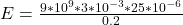## A charge of +3.0 µC is distributed uniformly along the circumference of a circle with a radius of 20 cm. How much external energy is require

Question

A charge of +3.0 µC is distributed uniformly along the circumference of a circle with a radius of 20 cm. How much external energy is required to bring a charge of 25µC from infinity to the center of the circle?

in progress 0
2 months 2021-07-31T21:20:52+00:00 2 Answers 0 views 0

The energy required is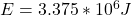Explanation:

From the question we are told that

The charge is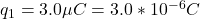The radius is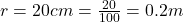The second charge is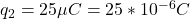Generally the energy required is mathematically represented as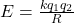Where k is the coulomb constant with a value of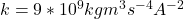Substituting values we have